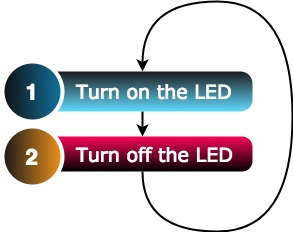### Intro to Arduino Coding and your First project!

Without loss of curiosity, let me tell you that your first project will be to blink an LED(which is inbuilt) using an Arduino.

Make sure you have the following with you,
1. An Arduino UNO or any other Arduino board
2. The Arduino IDE installed

Firstly, plug in the Arduino.
Open the Arduino IDE, and select the correct port (just letting the computer know, which USB point connects to the Arduino).

Before we jump into the coding bit, lets qualitatively discuss what we want the Arduino to do, and then convert the logic, into Arduino-understandable-code.

Our aim is to blink an LED.
Rephrasing this to a simpler instruction, we can say that we want to turn on an LED and then turn it OFF...and repeat this over and over.Unfortunately, the Arduino isn't smart enough to understand this level of abstraction. The Arduino would turn on the LED and turn it OFF in a fraction of a millisecond. So, it turns out we must explicitly tell the Arduino to wait for a second, before changing states.
And thats it!
Converting this to Arduino code would make it look like-

And thats the code you're looking for!
We'll talk about each line of code in a while. Before that, let's see some action on the Arduino.

```/*
* Whatever values you want to define
* that will be used later in the setup or the loop.
*/
int led=13; // so that the number 13 can be referred to as led

void setup() {
// put your setup code here, to run once:
pinMode(led, OUTPUT); //set the led pin to OUTPUT mode
}

void loop() {
// put your main code here, to run repeatedly:
digitalWrite(led, HIGH); //set led pin to HIGH state
delay(1000); // Wait for 1000ms
digitalWrite(led, LOW); //set led pin to LOW state
delay(1000); // Wait for 1000ms
}
```

.......and you should see a tiny LED on your board blinking at intervals of 1 second.In fact, you can also connect an external LED on pin 13 which would blink along with the inbuilt LED.

To do so, simply connect a 330/220 ohm resistor in series with the LED and connect it to pin 13 and GND.

By the way, this code can also be found in IDE under examples, just go to-
This is the "Hello World" of micro-controller programming.

Now, lets talk about Arduino coding in general.

Any code in here is generally divided into three parts.

1. Global declarations
2. Setup
3. Loop
Let's talk about each of these-
1. Global declarations- This is where you mention names and numbers that you want to use later in the code. For instance, if I wish to have a variable that is called 'count' and is initially equal to 0. I would write

```int count=0;
```

and now I can do whatever I want with 'count' in the setup or the loop. For instance if I had to increase the value of count by 1, I could directly write-

```count=count+1;
```

2. Setup- Whatever comes in between the curly brackets{} of setup, executes only once, when the Arduino is initially powered up. To re-run the setup part on your Arduino, you must press the on-board reset button.

3. Loop- Once the setup has been executed, whatever is written between the curly brackets{} of the loop function is executed over and over till the Arduino is powered off.

Having reached here, you now know how to blink an LED.
Below are some challenges to test your understanding so far and stregthen your coding skills.

2. Blink the LED only once.

3. Attach an LED to pin 7 and 'blink' it.

4. Attach an LED to any two digital pins(which means you don't connect the LED to GND) on the Arduino and blink it.